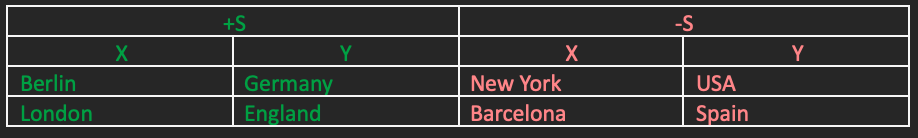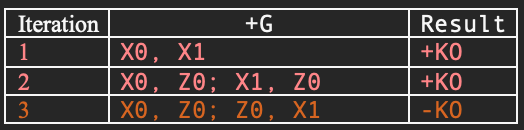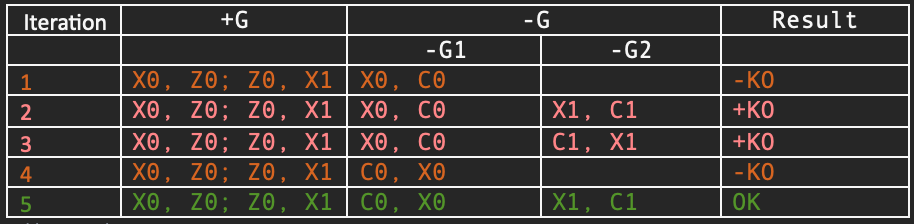# GILP: Symbolic Machine Learning Over Knowledge Graphs

Id Status Submission Date Publication Date Authorship
US17/546,450 Published 09 Dec 2021 18 May 2023 100%

Inductive Logic Programming (ILP) is a branch of machine learning (ML) that describe a family of algorithms for generating programs intended to return a given set of expected results.
GILP is an meta-rules ILP based method specifically optimized for minimizing remote queries on a graph database where information is stored.

## Problem Definition

Given a knowledge base (KB), a set of positive samples (+S) and negative samples (-S)1 , the ILP algorithm will generate a program (P) capable of returning at least +S while excluding -S when executed over KB. ILP could be depicted as a function <KB, +S, -S> → <KB, P> and it is ascribable as NP problem, though GILP increase efficiency by pruning out trees of impossible solutions. GILP is based on meta-rules that are checked via implementation specific query (Q). When a solution is found, the meta-rule is used within the Q variable instances to generate an implementation specific program P.

### Simple Example

Imagine to create a new relation that does not exists in the KB - for example the relation capitalOf out of the ConceptNet database, by solely combining existing relations and data. Purpose of the GILP task is to find efficiently the simplest pattern that satisfy +S while excluding -S. Consider then the predicate capitalOf(X, Y):The resulting program P will be:

capitalOf(X, Y) ← partOf(X, Y), isA(X, capital), isA(Y, country)

paraphrased in “X is the capital of Y when X is a capital and Y is a country and X is part of Y.”

## Meta-rule

Meta-rules represent the pattern of possible solutions in the search space. They are abstract structures isomorphic of the final program P. Meta-rules are the generic platform-independent models (PIM) to be transformed into platform-specific models (PSM).

PSM are linked to a specific information system, in the example above the PSM is transformed into Datalog, a simple logic programming language. Symbols for meta-rules are defined as following:

• R: relation variable. They are instantiated in P.
• X: final variable name.
• Z: temporary variable. Specific for joins.
• C: constant name. its value will be materialized in the final P.

Meta-symbols are differentiated by an incrementing suffix.
When considering the capitalOf example, the meta-rule defines the structure of the body of such rule. Given the body:

partOf(X, Y), isA(X, capital), isA(Y, country)

the generating meta-rule was:

R0(X0, X1), R1(X0, C0), R2(X1, C1)

where meta-symbols are substituted like in the Table.3.

Fundamental purpose of GILP is to find the most concise meta-rule in efficient way. Meta-rules are checked throughout the execution of Q, which are their PSM counterpart, and they run in the KB ecosystem. The Q meta-rule checking, is driven by the following macro tasks:
+G. Find the most unrestrictive (with least amount of computationally expensive joins) rule that matches +S.
-G. Gradually restrict the rule for pruning out -S while maintaining +S.
The successful meta-rule is the first that fulfill all constraints; therefore, the search is iterative process starting from the simplest meta-rule, to the most admissible complicated one. Q is generated by a given meta-rule under examination and return an answer corresponding to one of those types:
+KO: meta-rule does not match +S.
-KO: meta-rule matches +S but does not match -S.
OK: meta-rule successfully checked.
We will consider from now on, KBs with 2-arity relations only and we’ll omit, for simplicity, the meta-symbol R in the meta-rule generation.

This is the phase intended to find the relation pattern that matches +S. The most obvious meta-rule to start with is just [X0, X1] which corresponds to nodes in KB already linked with an existing relation R. Then it will try with meta-rules with progressively greater distance2 between the variable pair X0 and X1, like in this sequence:Table 1 - +G phase

The max distance of generated rules is configurable. The generated meta-rules must include all the possible permutations3 of the variable positions in the target KG.

The -G phase is performed over the set of +G meta-rule candidates that already have match +S (they returned -KO in +G phase). -G append restrictions over the variables already present in the candidate meta-rule.
Restrictions are defined as one or multiple relations that link a variable of type X or Z with one or more constants C. The algorithm for generating constraints is the same of +G but rather than generating combinations are permutations of X variables, it uses one X or Z variable within a constant C.
-G add more restrictions when none of in the current cycle (in Table.2 column -G1) has found an OK result. -G search will increase the number of restriction searches (for ex: the -G2) when a more restrictive discrimination is required for matching -S.
The process continues until a solution is found or all admissible restrictions (configurable) failed. In such case, -G is repeated but with the following +G meta-rule candidate.
Configuration parameters regulate the max number of vars, the max multi-hop level, and the max total number of restrictions.

### Skip Impossible Meta-rule Branches

Though computational complexity in GILP is categorized in the class of NP, the algorithm efficiently cut down branches of search spaces that can’t qualify as solutions.Table 3 - -G phase

## PSM Meta-rule Execution

The meta-rule selected for inquiry will be used to generate a platform specific query (Q).

ASP is a logic programming paradigm oriented to solve NP problems, and its declarative style is particularly suitable for didactical purposes. If you are not familiar with ASP, you can read some material about it.
Before running Q, +S and -S are injected (or made available) when running Q. The pos and neg predicates in Q are pointing respectively to +S and -S tuples.
Ex: pos(Berlin, Germany), neg(Barcelona, Spain).

Let’s take the initial example of finding P for the new relation capitalOf. A meta-rule returning OK is [X0, X1; X0, C0; X1, C1].
This meta-rule is used to show the entire process that bring a Q into P.
The corresponding ASP program4 for it is:

1. 1 {rel(R0,R1,R2,C0,C1)} 1 :- pos(X0,X1), R0(X0,X1), R1(X0,C0), R2(X1,C1)
2. :- pos(X0,X1), rel(R0,R1,R2,C0,C1), not5(R0(X0,X1), R1(X0,C0), R2(X1,C1))
3. :- rel(R0,R1,R2,C0,C1), R0(X0,X1), R1(X0,C0), R2(X1,C1), neg(X0,X1)

The lines are explained as follows:

1. Grounding. New predicate rel is generated as solution candidate. rel is the product of the given meta-rule variable matching +S. This line will generate as many solutions as the number of combinations of the enclosing variables. A solution is an independent set of predicates. In ASP this phase is called grounding.
2. +G phase. It prunes out all solutions that do not match all +S.
3. -G phase. It prunes out all solutions that do match with -S.

If all lines (1,2,3) return solutions, they can be used to generate P, and the ASP query Q returns OK result. If after line 2 there are solutions, but there are none after line 3, the result will be -KO.
In the case of successful OK, the rel predicate is materialized as:
rel(partOf, isA, isA, capital, country)The meta-rule is then rewritten within the materialized variables from Q, and it is transformed into the body of P:

[X0, X1; X0, C0; X1, C1] => partOf(X, Y), isA(X, capital), isA(Y, country)

Ultimately, P:
capitalOf(X, Y) ← partOf(X, Y), isA(X, capital), isA(Y, country)

### SparQL PSM

SparQL is a standard query language for graph databases.
There are 2 types of queries in this PSM:

• Check: it is used whether the given meta-rule is -KO, OK or +KO, without any return of
matched relations or constants.
• Bind: it is executed only after OK and returns relations and constants used for building the actual program that solve the positive and negative cases.
In SparQL, nodes are expressed as URI. For convenience we will express all node and relations with the same empty namespace :.

Check query example:

select (count(*) as ?pos_res) (sum(if(?neg = true, 1, 0)) as ?neg_res){
{
select *  {
:Berlin ?R0 :Germany. :Berlin ?R1 ?C0. :Germany ?R2 ?C1.
:London ?R0 :England. :London ?R1 ?C0. :England ?R2 ?C1.
}
}
bind(exists{
filter not exists{
:NY ?R0 :USA. :NY ?R1 ?C0. :USA ?R2 ?C1.
}
filter not exists{
:Barcelona ?R0 :Spain. :Barcelona ?R1 ?C0. :Spain ?R2 ?C1.
}
} as ?neg)
}


If the neg_res returned variable is greater than zero, then the answer will be OK. Else if the pos_res is greater than zero, then the answer will be +KO.
Otherwise, the answer will be -OK.
In case of OK result, the bind query will be performed for materializing required variables as:

select * {
{
select * {
:Berlin ?R0 :Germany. :Berlin ?R1 ?C1. :Germany ?R2 ?C2.
:London ?R0 :England. :London ?R1 ?C1. :England ?R2 ?C2.
}
}
filter not exists{
:NY ?R0 :USA. :NY ?R1 ?C0. :USA ?R2 ?C1.
}
filter not exists{
:Barcelona ?R0 :Spain. :Barcelona ?R1 ?C0. :Spain ?R2 ?C1.
}
} limit 5


The limit 5 (configurable) indicates that there is a max of 5 programs that might be useful to obtain. The different is just different constants (An). Out of the bind query we can obtain the variable materializations.

Final program P6 will be:

select ?X ?Y {
?X :partOf ?Y. ?X :isA ‘capital’. ?Y :isA ‘country’.
}


## Method Applicability

This method finds its natural applicability on graph based databases, but the same principle applies for any kind of database handling with discrete data.
The method assumes that predicates (or records in relational DB) have arity equals two as in the given example. It is easy to adapt this method for different arity, and that adaptation is omitted in the current documentation.
The distinction between meta-rule selection and Q execution phases brings practical benefits runtime optimization.
Meta-rule generation is not data-intensive while it requires to be implemented with high level programming languages, it is appropriate to run it outside the DB environment.
Q execution on the other hand, is data-intensive and must be as closest as possible to the data.

## State of the Art in ILP

ILP is an old field of research in ML and there are many algorithms. The one proposed here is attributable to the metarules family which already has several implementations (Emdeet al., 1983; De Raedt & Bruynooghe, 1992; Flener, 1996; Kietz & Wrobel, 1992; Wang et al.,2014; Muggleton et al., 2015; Cropper & Muggleton, 2016; Kaminski et al., 2018; Evans &Grefenstette, 2018; Bain & Srinivasan, 2018) in particular Popper ) but all of those methods do not treat the metarule generation, selection and optimization, which is actually a milestone of the method presented here.

1. Differently from gradient-based ML on which the loss function determines the discrepancy between expected and obtained results in continuous space (real numbers), the -E input is required because of the symbolic nature of the process.

2. Here, distance is defined by the number of joins necessary to link X0 with X1. In a graph, it could be represented as the shortest path between two nodes: X0 and X1.

3. We consider that not all relations in KB are symmetric therefore the meta-symbol position (subject or object in the 2-arity predicate) is relevant.

4. The generation is parametric to the meta-rule’s variables, and it is omitted here.

5. The relation not (negation as a failure) is reserved keyword in ASP and it is not a materialized predicate in the KB. If the predicate inside not is true, then it will fail and vice-versa.

6. The SparQL version is isomorphic to the initial Datalog script given as example, the two notations are equivalent.

Tags:

Updated: